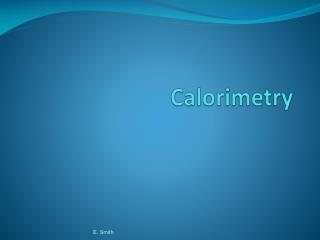DownloadDownload PresentationCalorimetry

# Calorimetry

Download Presentation## Calorimetry

- - - - - - - - - - - - - - - - - - - - - - - - - - - E N D - - - - - - - - - - - - - - - - - - - - - - - - - - -
##### Presentation Transcript

1. Calorimetry E. Smith

2. Calorimetry • The accurate and precise measurement of heat change for chemical and physical processes. • Calorimeter • An insulated device used to measure the absorption or release of heat in a chemical or physical process. E. Smith

3. E. Smith

4. Enthalphy…a Fancy Word for Heat • For systems with constant pressure, the heat content is the same as a property called enthalpy (H). • A change in heat at constant pressure is called a change in enthalpy (H) of a system. • At constant pressure • q = H = C x m x T E. Smith

5. Thermochemical Equations • A thermochemical equation expresses the heat needed or released in a chemical reaction. • Example of an exothermic reaction- • CaO(s) + H2O  Ca(OH)2 (s) + 65.2 kJ • Or the same equation can be expressed as • CaO(s) + H2O  Ca(OH)2 (s) H = - 65.2 kJ E. Smith

6. Endo- and Exo- • If the His negative (-) then the reaction is exothermic. • If the His positive (+) then the reaction is endothermic. • Knowing H will allow you to calculate the amount of heat released or gained in the reaction. E. Smith

7. Example • Gasohol contains ethanol (C2H5OH), which when burned reacts with excess oxygen to produce CO2 gas and water vapor. How much heat is released when 12.5 g of ethanol is burned? • C2H5OH (l) + O2 (g)  CO2 (g) + H2O (g) • H = -1235 kJ E. Smith

8. Answer to Example • First check if the equation is balanced! • C2H5OH(l) +3O2(g)  2CO2(g) + 3H2O(g) • 336 kJ of heat is released when 12.5 grams of C2H5OH is combusted with an excess of oxygen. The negative value tells you this is an exothermic reaction! E. Smith